## Analogy

#### Analogy

Direction: Select the related figure from the given alternatives.

1. Find the question mark ? figure from answer figure.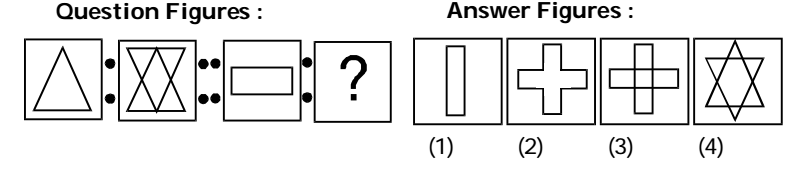1. From first figure to second figure one inverted design is added.

##### Correct Option: C

From first figure to second figure one inverted design is added.

1. Find the question mark ? figure from answer figure.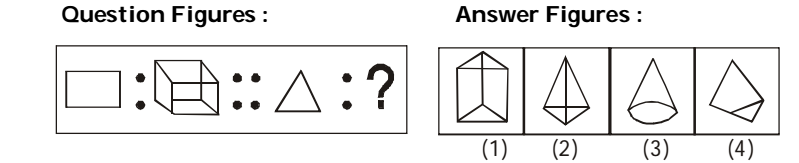1. From first figure to second figure the rectangle becomes cuboid. [3D-figure]

##### Correct Option: A

From first figure to second figure the rectangle becomes cuboid. [3D-figure]

1. Find the question mark ? figure from answer figure.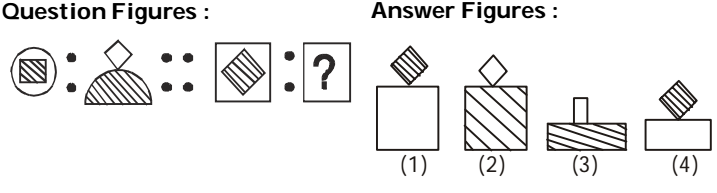1. From first figure to second figure half part of outer design moves downward and becomes shaded while the inner design moves upward after being rotated through 450 and becoming unshaded.

##### Correct Option: C

From first figure to second figure half part of outer design moves downward and becomes shaded while the inner design moves upward after being rotated through 450 and becoming unshaded.

1. Find the question mark ? figure from answer figure.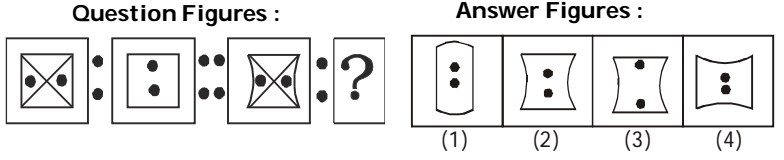1. From first figure to second figure the diagonals are deleted and both the dots are arranged vertically.

##### Correct Option: B

From first figure to second figure the diagonals are deleted and both the dots are arranged vertically.

1. Find the question mark ? figure from answer figure.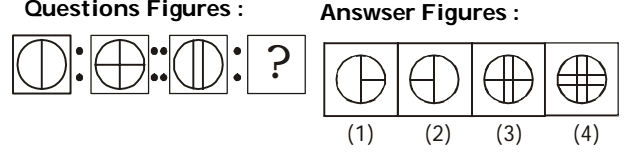1. From first figure to second figure one horizontal line segment is added. Similarly, from third figure to answer figure two horizontal line segments would be added.

##### Correct Option: D

From first figure to second figure one horizontal line segment is added. Similarly, from third figure to answer figure two horizontal line segments would be added.